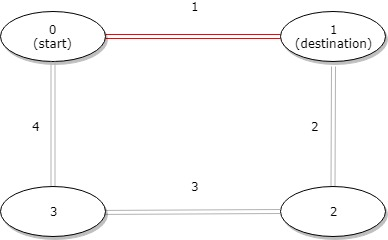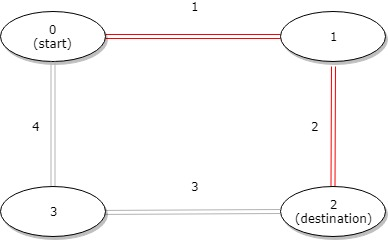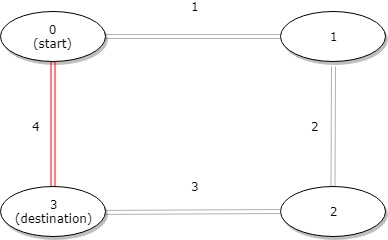1184. Distance Between Bus Stops

A bus has `n` stops numbered from `0` to `n - 1` that form a circle. We know the distance between all pairs of neighboring stops where `distance[i]` is the distance between the stops number `i` and `(i + 1) % n`.

The bus goes along both directions i.e. clockwise and counterclockwise.

Return the shortest distance between the given `start` and `destination` stops.

Example 1:```Input: distance = [1,2,3,4], start = 0, destination = 1
Output: 1
Explanation: Distance between 0 and 1 is 1 or 9, minimum is 1.```

Example 2:```Input: distance = [1,2,3,4], start = 0, destination = 2
Output: 3
Explanation: Distance between 0 and 2 is 3 or 7, minimum is 3.
```

Example 3:```Input: distance = [1,2,3,4], start = 0, destination = 3
Output: 4
Explanation: Distance between 0 and 3 is 6 or 4, minimum is 4.
```

Constraints:

• `1 <= n <= 10^4`
• `distance.length == n`
• `0 <= start, destination < n`
• `0 <= distance[i] <= 10^4`

1184. Distance Between Bus Stops
``````struct Solution;

impl Solution {
fn distance_between_bus_stops(distance: Vec<i32>, mut start: i32, mut destination: i32) -> i32 {
let n: usize = distance.len();
let mut left = 0;
let mut right = 0;
if destination < start {
std::mem::swap(&mut start, &mut destination);
}
for i in 0..n {
let j = start as usize + i;
if j < destination as usize {
left += distance[j % n];
} else {
right += distance[j % n];
}
}
i32::min(left, right)
}
}

#[test]
fn test() {
let distance = vec![1, 2, 3, 4];
let start = 0;
let destination = 1;
let res = 1;
assert_eq!(
Solution::distance_between_bus_stops(distance, start, destination),
res
);
let distance = vec![1, 2, 3, 4];
let start = 0;
let destination = 1;
let res = 1;
assert_eq!(
Solution::distance_between_bus_stops(distance, start, destination),
res
);
let distance = vec![1, 2, 3, 4];
let start = 0;
let destination = 2;
let res = 3;
assert_eq!(
Solution::distance_between_bus_stops(distance, start, destination),
res
);
let distance = vec![1, 2, 3, 4];
let start = 0;
let destination = 3;
let res = 4;
assert_eq!(
Solution::distance_between_bus_stops(distance, start, destination),
res
);
let distance = vec![3, 6, 7, 2, 9, 10, 7, 16, 11];
let start = 6;
let destination = 2;
let res = 28;
assert_eq!(
Solution::distance_between_bus_stops(distance, start, destination),
res
);
}
``````